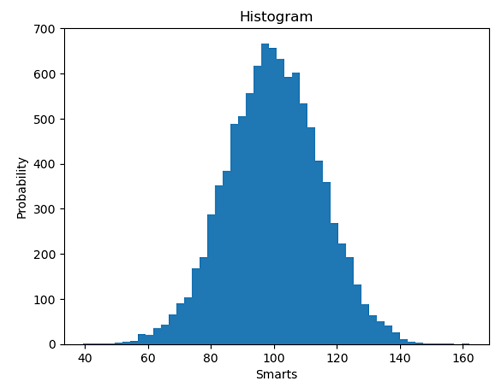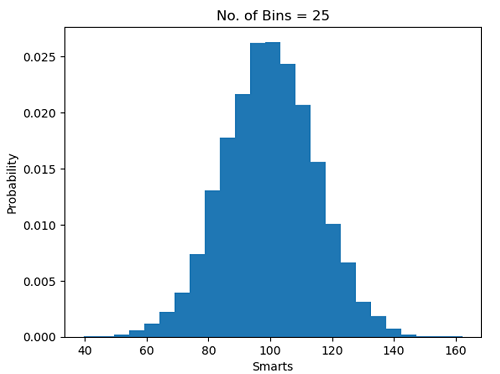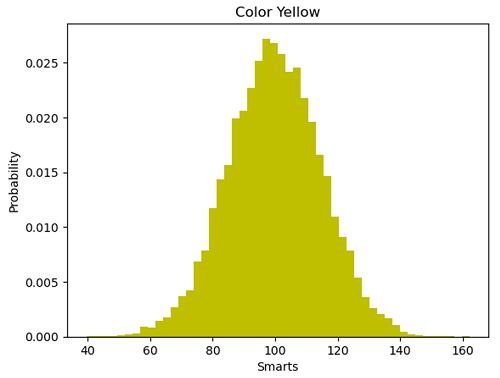# Python | Histogram Plotting

Python | Histogram Plotting: In this article, we are going to learn how to create Histogram plots in Python?
Submitted by Anuj Singh, on July 16, 2020

A histogram is a graphical technique or a type of data representation using bars of different heights such that each bar group's numbers into ranges (bins or buckets). Taller the bar higher the data falls in that bin. A Histogram is one of the most used techniques in data visualization and therefore, matplotlib has provided a function matplotlib.pyplot.hist() for plotting histograms.

The following example shows an illustration of the histogram.

```plt.hist(x, 50)
#Histogram with number of bins = 50
``````plt.hist(x, 25, density=1)
#Histogram with number of bins = 25
#Histogram with density = 1
``````plt.hist(x, 50, color='y')
#Histogram with number of bins = 50
#Histogram with color = yellow
```## Python code for histogram plotting

```import matplotlib.pyplot as plt
import numpy as np

# random data generation
mu, sigma = 100, 15
x = mu + sigma * np.random.randn(10000)

# Histogram of the Data
plt.figure()
plt.hist(x, 50)
plt.xlabel('Smarts')
plt.ylabel('Probability')
plt.title('Histogram')
plt.show()

plt.figure()
plt.hist(x, 25, density=1)
plt.xlabel('Smarts')
plt.ylabel('Probability')
plt.title('No. of Bins = 25')
plt.show()

plt.figure()
plt.hist(x, 50, density=1, facecolor='y')
plt.xlabel('Smarts')
plt.ylabel('Probability')
plt.title('Color Yellow')
plt.show()
```

Output:

```Output is as figure
```